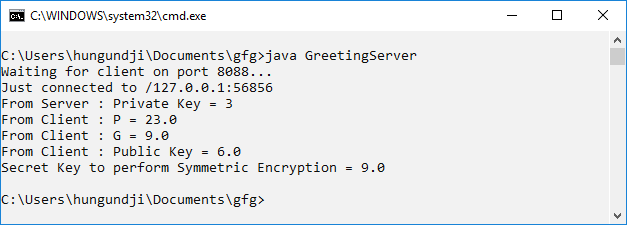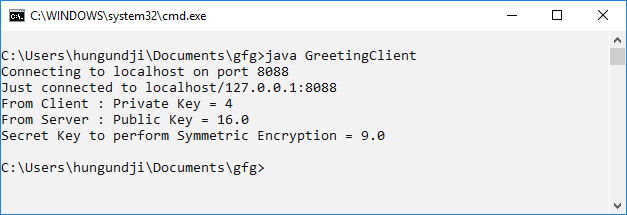Related Articles

# Java Implementation of Deffi-Hellman Algorithm between Client and Server

• Difficulty Level : Medium
• Last Updated : 18 Oct, 2018

Program to implement Deffi-Hellman Algorithm in Client-Server Fashion.

Prerequisite: Server Socket Programming, Diffie-Hellman algorithm

The Diffie Hellman Algorithm is being used to establish a shared secret that can be used for secret communications while exchanging data over a public network.

In the below program, the client will share the value of,, and public key. Whereas, the server will accept the values and calculate its public keyand send it to the client.

Both Client and Server will calculate the secret key for symmetric encryption by using the public key.

Program 1: Server Program

 `import` `java.net.*;``import` `java.io.*;`` ` `public` `class` `GreetingServer {``    ``public` `static` `void` `main(String[] args) ``throws` `IOException``    ``{``        ``try` `{``            ``int` `port = ``8088``;`` ` `            ``// Server Key``            ``int` `b = ``3``;`` ` `            ``// Client p, g, and key``            ``double` `clientP, clientG, clientA, B, Bdash;``            ``String Bstr;`` ` `            ``// Established the Connection``            ``ServerSocket serverSocket = ``new` `ServerSocket(port);``            ``System.out.println(``"Waiting for client on port "` `+ serverSocket.getLocalPort() + ``"..."``);``            ``Socket server = serverSocket.accept();``            ``System.out.println(``"Just connected to "` `+ server.getRemoteSocketAddress());`` ` `            ``// Server's Private Key``            ``System.out.println(``"From Server : Private Key = "` `+ b);`` ` `            ``// Accepts the data from client``            ``DataInputStream in = ``new` `DataInputStream(server.getInputStream());`` ` `            ``clientP = Integer.parseInt(in.readUTF()); ``// to accept p``            ``System.out.println(``"From Client : P = "` `+ clientP);`` ` `            ``clientG = Integer.parseInt(in.readUTF()); ``// to accept g``            ``System.out.println(``"From Client : G = "` `+ clientG);`` ` `            ``clientA = Double.parseDouble(in.readUTF()); ``// to accept A``            ``System.out.println(``"From Client : Public Key = "` `+ clientA);`` ` `            ``B = ((Math.pow(clientG, b)) % clientP); ``// calculation of B``            ``Bstr = Double.toString(B);`` ` `            ``// Sends data to client``            ``// Value of B``            ``OutputStream outToclient = server.getOutputStream();``            ``DataOutputStream out = ``new` `DataOutputStream(outToclient);`` ` `            ``out.writeUTF(Bstr); ``// Sending B`` ` `            ``Bdash = ((Math.pow(clientA, b)) % clientP); ``// calculation of Bdash`` ` `            ``System.out.println(``"Secret Key to perform Symmetric Encryption = "``                               ``+ Bdash);``            ``server.close();``        ``}`` ` `        ``catch` `(SocketTimeoutException s) {``            ``System.out.println(``"Socket timed out!"``);``        ``}``        ``catch` `(IOException e) {``        ``}``    ``}``}`

Program 2: Client Program

 `import` `java.net.*;``import` `java.io.*;`` ` `public` `class` `GreetingClient {``    ``public` `static` `void` `main(String[] args)``    ``{``        ``try` `{``            ``String pstr, gstr, Astr;``            ``String serverName = ``"localhost"``;``            ``int` `port = ``8088``;`` ` `            ``// Declare p, g, and Key of client``            ``int` `p = ``23``;``            ``int` `g = ``9``;``            ``int` `a = ``4``;``            ``double` `Adash, serverB;`` ` `            ``// Established the connection``            ``System.out.println(``"Connecting to "` `+ serverName``                               ``+ ``" on port "` `+ port);``            ``Socket client = ``new` `Socket(serverName, port);``            ``System.out.println(``"Just connected to "``                               ``+ client.getRemoteSocketAddress());`` ` `            ``// Sends the data to client``            ``OutputStream outToServer = client.getOutputStream();``            ``DataOutputStream out = ``new` `DataOutputStream(outToServer);`` ` `            ``pstr = Integer.toString(p);``            ``out.writeUTF(pstr); ``// Sending p`` ` `            ``gstr = Integer.toString(g);``            ``out.writeUTF(gstr); ``// Sending g`` ` `            ``double` `A = ((Math.pow(g, a)) % p); ``// calculation of A``            ``Astr = Double.toString(A);``            ``out.writeUTF(Astr); ``// Sending A`` ` `            ``// Client's Private Key``            ``System.out.println(``"From Client : Private Key = "` `+ a);`` ` `            ``// Accepts the data``            ``DataInputStream in = ``new` `DataInputStream(client.getInputStream());`` ` `            ``serverB = Double.parseDouble(in.readUTF());``            ``System.out.println(``"From Server : Public Key = "` `+ serverB);`` ` `            ``Adash = ((Math.pow(serverB, a)) % p); ``// calculation of Adash`` ` `            ``System.out.println(``"Secret Key to perform Symmetric Encryption = "``                               ``+ Adash);``            ``client.close();``        ``}``        ``catch` `(Exception e) {``            ``e.printStackTrace();``        ``}``    ``}``}`

Use javac to Compile the programs, and open two console/terminal to run the system

Output:
In the first console run the server program, it will wait for the client’s connection. As soon as client is connected results will popupIn the second console, run the client’s programAttention reader! Don’t stop learning now. Get hold of all the important CS Theory concepts for SDE interviews with the CS Theory Course at a student-friendly price and become industry ready.

My Personal Notes arrow_drop_up